Question

# a) Using the EDD (earliest due date) decision rule for sequencing the jobs, the order is...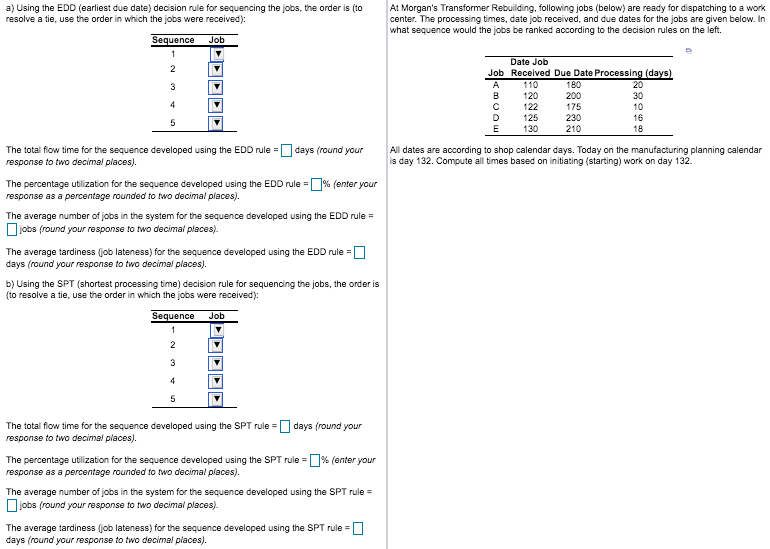a)

Using EDD (earliest due date), we will sequence jobs with earliest due date first and then so on and so forth.

 Sequence Job Due date 1 C 175 2 A 180 3 B 200 4 E 210 5 D 230

We will calculate other parameters as below

 Sequence Job Processing (Days) Flow Time Due date 1 C 10 10 175 2 A 20 30 180 3 B 30 60 200 4 E 18 78 210 5 D 16 94 230 Total 94 272

Total flow time for sequence using EDD rule is 272 days.

Percentage utilization for sequence developed using EDD rule is 94/ 272 = 0.3456 or 34.56%

Average number of jobs in the system for sequence developed using EDD rule is 272/ 94 = 2.89 jobs.

For calculating tardiness, we will have to consider that starting work day is 132 and calculated tardiness as Completion date - Due date (0 if negative)

 Sequence Job Processing (Days) Date Job Received Completion date Due date Tardiness 1 C 10 122 132 + 10 = 142 175 0 (142 - 175 is negative) 2 A 20 110 142 + 20 = 162 180 0 (162 - 180 is negative) 3 B 30 120 162 + 30 = 192 200 0 (192 - 200 is negative) 4 E 18 130 192 + 18 = 210 210 0 5 D 16 125 210 + 16 = 226 230 0 (226- 230 is negative) Total 94 0

Hence, Average tardiness (or job lateness)  for sequence developed using EDD rule is 0/5 = 0 days.

b)

Using SPT (shortest processing time) rule, we will sequence shortest time job first and so on and so forth.

 Sequence Job Processing (Days) 1 C 10 2 D 16 3 E 18 4 A 20 5 B 30

We will calculate other parameters as below

 Sequence Job Processing (Days) Flow time 1 C 10 10 2 D 16 26 3 E 18 44 4 A 20 64 5 B 30 94 Total 94 238

Total flow time for the sequence developed using SPT rule = 238 days

Percentage utilization for the sequence developed using SPT rule = 94/ 238 = 0.3950 or 39.50%

Average number of jobs in the system for the sequence developed using SPT rule = 238/ 94 = 2.53 jobs

For calculating tardiness, we will have to consider that starting work day is 132 and calculated tardiness as Completion date - Due date (0 if negative)

 Sequence Job Processing (Days) Date Job Received Completion date Due date Tardiness 1 C 10 122 132 + 10 = 142 175 0 (142 - 175 is negative) 2 D 16 125 142 + 16 = 158 230 0 (158 - 230 is negative) 3 E 18 130 158 + 18 = 176 210 0 (176 - 210 is negative) 4 A 20 110 176 + 20 = 196 180 16 (196 - 180) 5 B 30 120 196 + 30 = 226 200 26 (226- 200) Total 42

Average tardiness using SPT rule = Total tardiness/ number of jobs = 42/ 5 = 8.4 days.

If you liked my answer, please give like/ thumbs up. Thank you!

#### Earn Coins

Coins can be redeemed for fabulous gifts.

Similar Homework Help Questions
• ### a) Using the EDD (earliest due date) decision rule for sequencing the jobs, the order is...a) Using the EDD (earliest due date) decision rule for sequencing the jobs, the order is (to resolve a tie, use the order in which the jobs were received): At Morgan's Transformer Rebuilding, following jobs (below) are ready for dispatching to a work center. The processing times, date job received, and due dates for the jobs are given below. In what sequence would the jobs be ranked according to the decision rules on the left Sequence Job 1 Date Job...

• ### ​a) Using the SPT​ (shortest processing​ time) decision rule for sequencing the​ jobs, the order is​...

​a) Using the SPT​ (shortest processing​ time) decision rule for sequencing the​ jobs, the order is​ (to resolve a​ tie, use the order in which the jobs were​ received): Sequence Job 1 ▼ N L M K O 2 ▼ K O L M N 3 ▼ K N L O M 4 ▼ N L M O K 5 ▼ N O K M L The total flow time for the sequence developed using the SPT rule​ = nothing...

• ### a) Using the FCFS (first come, first served) decision e for sequencing the jobs, the order...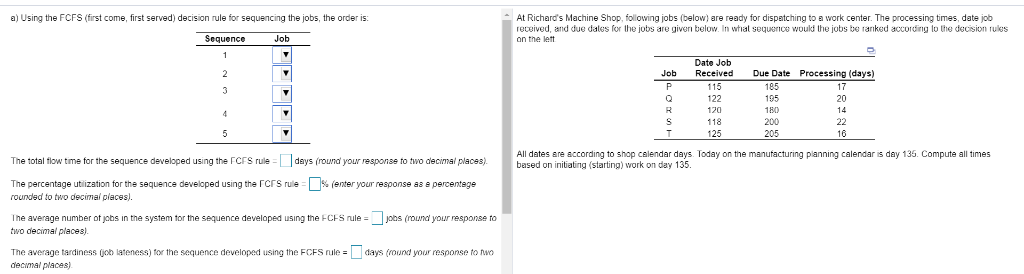a) Using the FCFS (first come, first served) decision e for sequencing the jobs, the order is t Richard's Machine Shop, following jobs (below) are ready for dispatching to a work center. The processing times, date job recuived, and due dales for the jobs a ranked according lo the decision rukes given below whal sequence would the jobs b Sequence Job Date Job Processing (days) Job Received D 105 122 195 20 180 120 205 25 I times d daysloday...

• ### Using the FCFS (first come, first served) decision rule for sequencing the jobs, the order is:...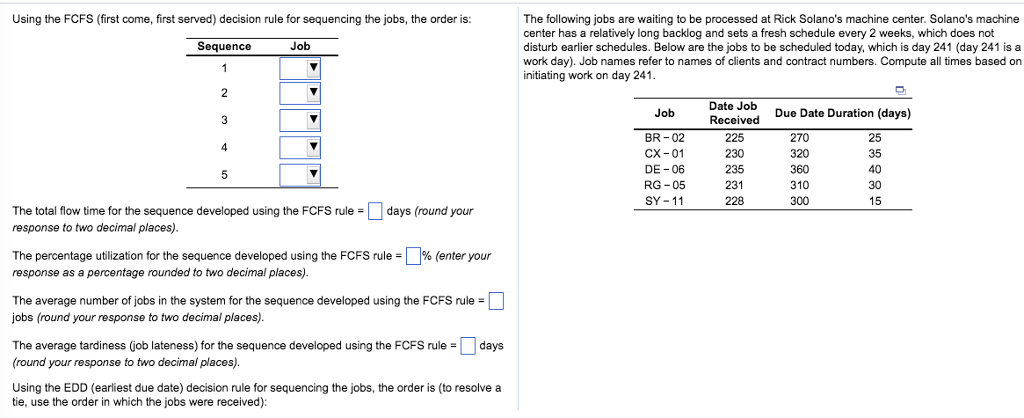Using the FCFS (first come, first served) decision rule for sequencing the jobs, the order is: The following jobs are waiting to be processed at Rick Solano's machine center. Solano's machine center has a relatively long backlog and sets a fresh schedule every 2 weeks, which does not disturb earlier schedules. Below are the jobs to be scheduled today, which is day 241 (day 241 is a work day). Job names refer to names of clients and contract numbers. Compute...

• ### decimal places). An Alabama lumberyard has four jobs on order, as shown in the following table....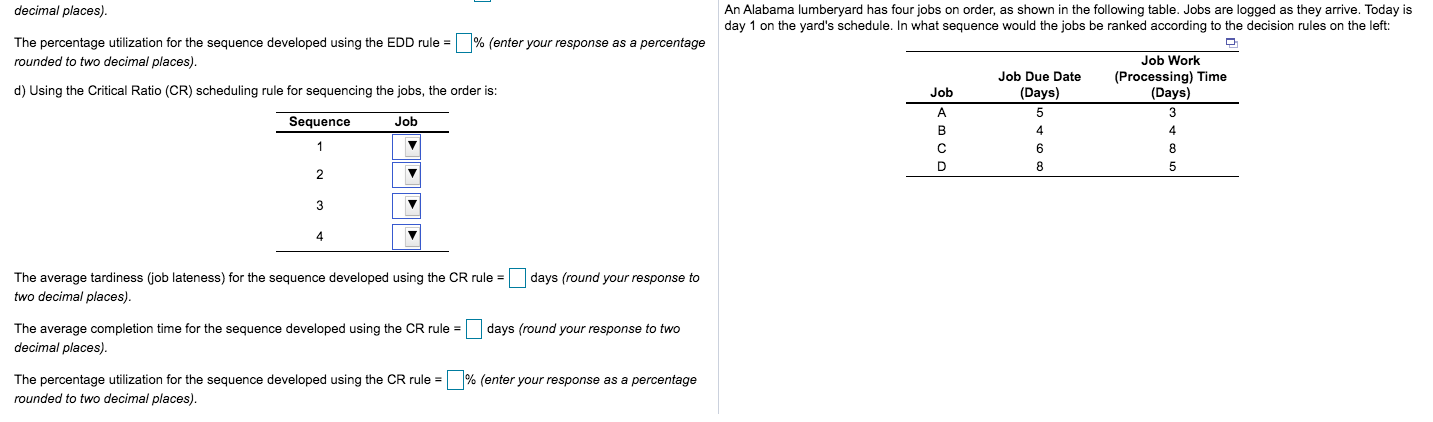decimal places). An Alabama lumberyard has four jobs on order, as shown in the following table. Jobs are logged as they arrive. Today is day 1 on the yard's schedule. In what sequence would the jobs be ranked according to the decision rules on the left: % (enter your response as a percentage The percentage utilization for the sequence developed using the EDD rule = rounded to two decimal places). Job Work (Processing) Time (Days) Job Due Date (Days) d)...

• ### a) Using the FCFS​ (first come, first served​ ) decision rule for sequencing the​ jobs, the...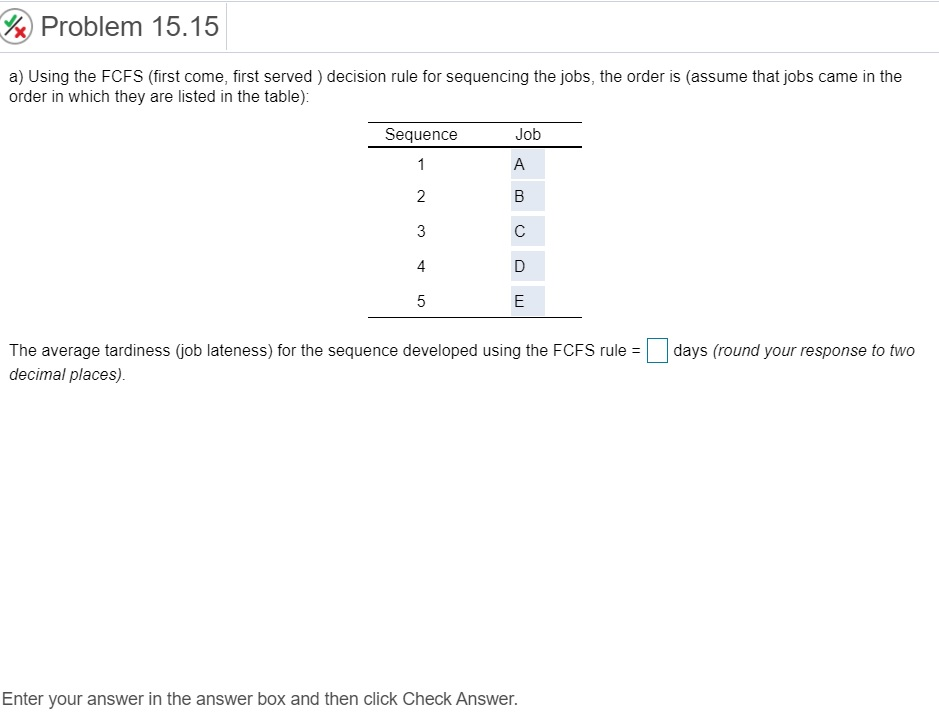a) Using the FCFS​ (first come, first served​ ) decision rule for sequencing the​ jobs, the order is​ (assume that jobs came in the order in which they are listed in the​ table): Sequence Job 1 A 2 B 3 C 4 D 5 E The average tardiness​ (job lateness) for the sequence developed using the FCFS rule​ = ___ days ​(round your response to two decimal​ places). The following jobs are waiting to be processed at the same machine...

• ### ABC Enterprises has five jobs that must be scheduled for production at a work center. Below...

ABC Enterprises has five jobs that must be scheduled for production at a work center. Below are the processing times and due dates for each job.                                     Job Processing Time (in days) Due Date (days from today) 1 7 14 2 5 21 3 3 10 4 2 7 5 6 9                                                                                                                            Schedule the jobs by sequencing them using the Earliest Due Date (EDD) priority rule. What is the average flow time? Show your Work and round your...

• ### Processing times and due dates for five jobs are shown in the table below. Assume the...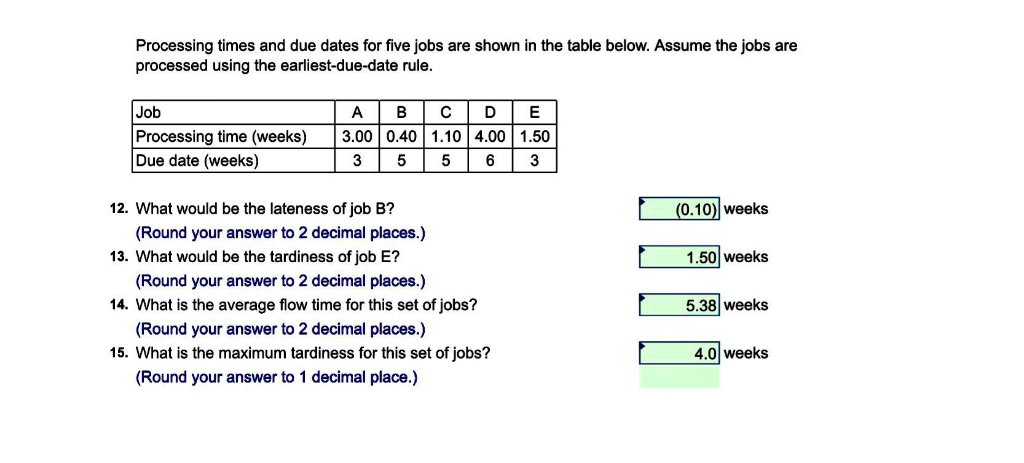Processing times and due dates for five jobs are shown in the table below. Assume the jobs are processed using the earliest-due-date rule Job Processing time (weeks) 3.00 0.40 1.10 4.00 1.50 Due date (weeks) 3 556 3 12. What would be the lateness of job B? 13. What would be the tardiness of job E? 14. What is the average flow time for this set of jobs? 15. What is the maximum tardiness for this set of jobs? (0.10...

• ### Problem 18 Question Help Estimated Machine Time (hours) Time Since Order Due Date Arrived (hours ago)...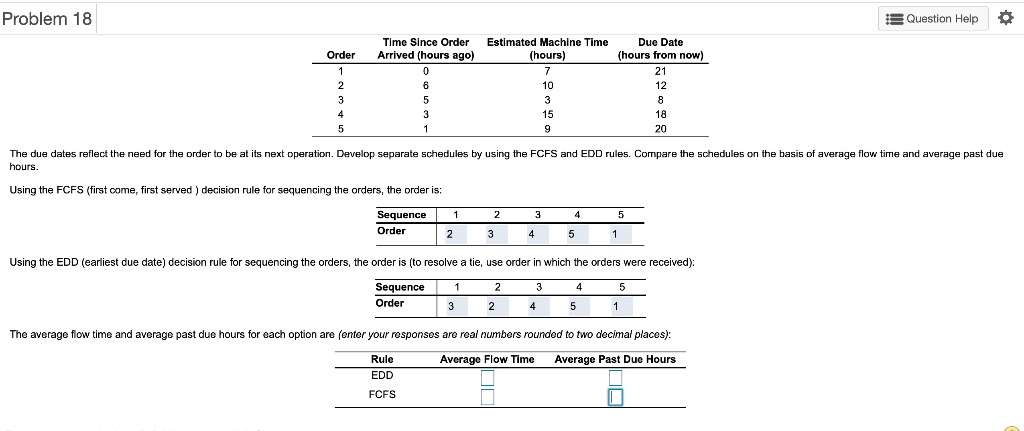Problem 18 Question Help Estimated Machine Time (hours) Time Since Order Due Date Arrived (hours ago) (hours from now) Order 1 0 2* 10 12 3 5 8 3 18 20 The due dates reflect the need for the order to be hours t its next operation. Develop separate schedules by using the FCFS and EDD rules. Compare the schedules on the basis of average flow time and average past due Using the FCFS (first come, first served) decision rule...

• ### What would be the sequence of the following jobs if you did them in Earliest Due...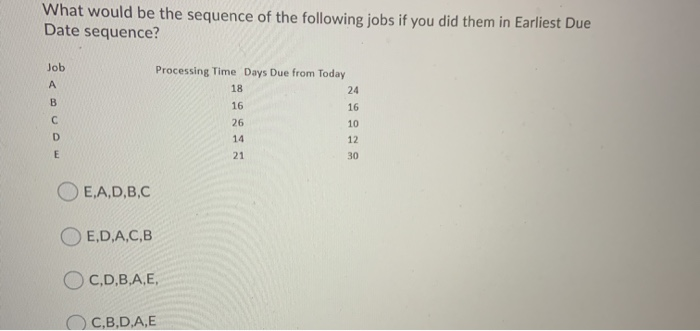What would be the sequence of the following jobs if you did them in Earliest Due Date sequence? Job A B Processing Time Days Due from Today 18 24 16 16 26 10 14 12 21 30 С D E OE,A,D,B,C E,D,A,C,B OC,D,B,A,E, C.B.D.A,E Question 6 (10 points) You have 5 jobs to do. What would be the sequence if you chose to do a Shortest Processing Time Approach? Job Processing Time Days Due from Today А 24 30 B...